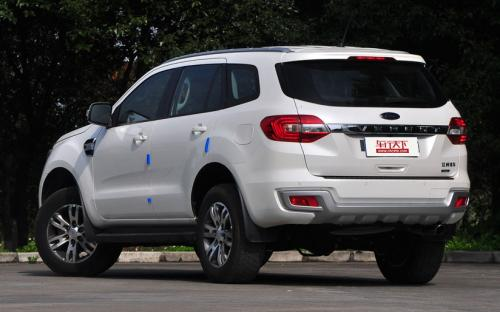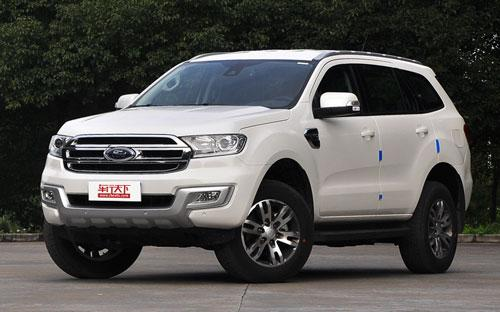江铃福特 撼路者6 种颜色可选2016款最低售价：26.58 万元起

4892(mm)1862(mm)1837(mm)配置亮点：
• 胎压监测装置

• ISOFIX儿童座椅接口

• 车身稳定控制(ESC/ESP/DSC等)

• 电动天窗

• 定速巡航

• 后倒车雷达

• 真皮座椅

• GPS导航系统

• 氙气大灯

• 后视镜加热

2016款 2.0T 汽油自动四驱菁英版 (94张)
• 2016款 2.0T 汽油自动四驱菁英版 (94张)
• 2016款 2.0T 汽油自动四驱豪华版 (111张)江铃福特 撼路者 在售车型

排量 车型 厂商指导价 本地最低报价 购车工具
2.0T 2.2T 柴油手动两驱菁英版 柴油 6挡手动 26.58万 询底价+对比
2.0T 汽油自动两驱菁英版 6挡手自一体 27.38万 询底价+对比
2.0T 汽油自动四驱菁英版 6挡手自一体 28.58万 询底价+对比
2.0T 汽油自动两驱豪华版 6挡手自一体 30.78万 询底价+对比
2.2T 柴油手动四驱豪华版 柴油 6挡手动 30.98万 询底价+对比
2.0T 汽油自动四驱豪华版 6挡手自一体 31.88万 询底价+对比
2.0T 汽油自动四驱旗舰版 6挡手自一体 36.08万 询底价+对比
2.2T 2.2T 柴油手动两驱菁英版 柴油 6挡手动 26.58万 询底价+对比
2.0T 汽油自动两驱菁英版 6挡手自一体 27.38万 询底价+对比
2.0T 汽油自动四驱菁英版 6挡手自一体 28.58万 询底价+对比
2.0T 汽油自动两驱豪华版 6挡手自一体 30.78万 询底价+对比
2.2T 柴油手动四驱豪华版 柴油 6挡手动 30.98万 询底价+对比
2.0T 汽油自动四驱豪华版 6挡手自一体 31.88万 询底价+对比
2.0T 汽油自动四驱旗舰版 6挡手自一体 36.08万 询底价+对比

江铃福特 撼路者 经销商

查看更多 >>
更多 >>

本地降价排名

江铃福特 撼路者 动力加速

撼路者 0-100公里加速时间分布在 0.0-秒 属于 超跑级

动力级别 加速时间 车型

江铃福特 撼路者 视频

江铃福特 撼路者 新闻资讯

上海车展新撼路者巅峰再起

即将上市 超过9044次关注

4月5日，新福特撼路者在“世界屋脊”珠峰大本营宣布上市，总共推出九款车型，官方指导价为26.58-36.08万元。在原车型越野性、安全性全方位领先的基础上，新福特撼路...

江铃福特撼路者上市 售价26.58-36.08万元

上市新车 超过8314次关注

10月30日，江铃福特旗下的硬派SUV撼路者正式上市。新车采用了大尺寸的镀铬格栅，造型十分粗犷；新车配备全时四驱系统，搭载2.0LGTDi汽油发动机和2.2LTDCi柴油发动机...

金牛座等 福特下半年将在国内推5款新车

行业动态 超过8290次关注

福特官方称，将在2015下半年投放5款新车型，包括福特金牛座、撼路者、全新探险者、新款福克斯和新款福克斯ST，助力福特在华2015下半年销量增长随着下半年为中国市场...

江铃福特撼路者新消息 将于10月上市

国产新车 超过6383次关注

日前我们获悉，江铃福特撼路者将于4月的上海国际车展再度亮相，另据国内媒体报道，新车将于今年10月份正式上市。根据之前了解，这款7座硬派SUV预计售价为30-45万元...

预计9月上市 疑似国产撼路者柴油版谍照

道听途说 超过5442次关注

据悉，我们获得了一组福特撼路者的国内测试谍照，新车前脸依然采用了六边形的前进气格栅，前大灯采用了熏黑处理；中控台整体采用了全黑的配色，座椅为浅色织物面料...

预售26.98万起 福特撼路者10月30日上市

即将上市 超过8854次关注

据官方获悉，江铃福特撼路者将会在10月30日正式上市，作为一款中型SUV，其主打硬派越野风格。造型非常粗犷，搭载2.0T汽油发动机和2.2T柴油发动机，共推出7款车型，...

江铃福特撼路者 有望于2015年9月上市

国产新车 超过4081次关注

近日，国内媒体获悉，江铃福特撼路者预计将于2015年9月份前后正式上市。新车采用7座布局设计，并定位于中型SUV。据报道称，这款车预计售价为30-45万元。

猜你喜欢

﻿
• 快速找车
• 选择品牌
• 选择品牌
• A  奥迪
• A  阿斯顿·马丁
• A  阿尔法·罗密欧
• B  宝沃
• B  布加迪
• B  巴博斯
• B  保时捷
• B  宾利
• B  奔驰
• B  宝马
• B  本田
• B  别克
• B  标致
• B  比亚迪
• B  宝骏
• B  北汽制造
• B  北汽新能源
• B  北汽幻速
• B  北汽威旺
• B  北京汽车
• B  奔腾
• B  北汽绅宝
• C  长安
• C  长安商用
• C  长城
• C  昌河
• D  大众
• D  道奇
• D  DS
• D  东南
• D  东风风神
• D  东风风行
• D  东风小康
• D  东风风度
• D  东风
• F  福特
• F  丰田
• F  菲亚特
• F  法拉利
• F  福田
• F  福迪
• F  福汽启腾
• G  观致
• G  广汽传祺
• G  广汽吉奥
• G  GMC
• H  红旗
• H  汉腾汽车
• H  哈弗
• H  哈飞
• H  海格
• H  海马
• H  华颂
• H  黄海
• H  华泰
• H  恒天
• J  吉利汽车
• J  捷豹
• J  Jeep
• J  江淮
• J  江铃
• J  金杯
• J  九龙
• J  金旅
• K  凯翼
• K  凯迪拉克
• K  克莱斯勒
• K  科尼塞克
• K  卡威
• K  开瑞
• L  路虎
• L  林肯
• L  劳斯莱斯
• L  兰博基尼
• L  雷克萨斯
• L  铃木
• L  雷诺
• L  理念
• L  力帆
• L  莲花汽车
• L  猎豹
• L  路特斯
• L  陆风
• M  马自达
• M  MG
• M  MINI
• M  玛莎拉蒂
• M  摩根
• M  迈凯轮
• N  纳智捷
• O  欧宝
• O  讴歌
• O  欧朗
• Q  奇瑞
• Q  起亚
• Q  启辰
• R  日产
• R  荣威
• R  瑞麒
• S  三菱
• S  斯威汽车
• S  萨博
• S  smart
• S  斯柯达
• S  斯巴鲁
• S  思铭
• S  双龙
• S  上汽大通
• S  双环
• T  特斯拉
• T  腾势
• W  沃尔沃
• W  五菱汽车
• W  五十铃
• W  威兹曼
• W  威麟
• X  现代
• X  雪佛兰
• X  雪铁龙
• X  西雅特
• Y  一汽
• Y  英菲尼迪
• Y  英致
• Y  依维柯
• Y  野马汽车
• Y  永源
• Z  众泰
• Z  中华
• Z  中兴
• Z  知豆
• 选择车系
• 选择车系
• 车型对比
• 选择品牌
• 选择品牌
• A  奥迪
• A  阿斯顿·马丁
• A  阿尔法·罗密欧
• B  宝沃
• B  布加迪
• B  巴博斯
• B  保时捷
• B  宾利
• B  奔驰
• B  宝马
• B  本田
• B  别克
• B  标致
• B  比亚迪
• B  宝骏
• B  北汽制造
• B  北汽新能源
• B  北汽幻速
• B  北汽威旺
• B  北京汽车
• B  奔腾
• B  北汽绅宝
• C  长安
• C  长安商用
• C  长城
• C  昌河
• D  大众
• D  道奇
• D  DS
• D  东南
• D  东风风神
• D  东风风行
• D  东风小康
• D  东风风度
• D  东风
• F  福特
• F  丰田
• F  菲亚特
• F  法拉利
• F  福田
• F  福迪
• F  福汽启腾
• G  观致
• G  广汽传祺
• G  广汽吉奥
• G  GMC
• H  红旗
• H  汉腾汽车
• H  哈弗
• H  哈飞
• H  海格
• H  海马
• H  华颂
• H  黄海
• H  华泰
• H  恒天
• J  吉利汽车
• J  捷豹
• J  Jeep
• J  江淮
• J  江铃
• J  金杯
• J  九龙
• J  金旅
• K  凯翼
• K  凯迪拉克
• K  克莱斯勒
• K  科尼塞克
• K  卡威
• K  开瑞
• L  路虎
• L  林肯
• L  劳斯莱斯
• L  兰博基尼
• L  雷克萨斯
• L  铃木
• L  雷诺
• L  理念
• L  力帆
• L  莲花汽车
• L  猎豹
• L  路特斯
• L  陆风
• M  马自达
• M  MG
• M  MINI
• M  玛莎拉蒂
• M  摩根
• M  迈凯轮
• N  纳智捷
• O  欧宝
• O  讴歌
• O  欧朗
• Q  奇瑞
• Q  起亚
• Q  启辰
• R  日产
• R  荣威
• R  瑞麒
• S  三菱
• S  斯威汽车
• S  萨博
• S  smart
• S  斯柯达
• S  斯巴鲁
• S  思铭
• S  双龙
• S  上汽大通
• S  双环
• T  特斯拉
• T  腾势
• W  沃尔沃
• W  五菱汽车
• W  五十铃
• W  威兹曼
• W  威麟
• X  现代
• X  雪佛兰
• X  雪铁龙
• X  西雅特
• Y  一汽
• Y  英菲尼迪
• Y  英致
• Y  依维柯
• Y  野马汽车
• Y  永源
• Z  众泰
• Z  中华
• Z  中兴
• Z  知豆
• 选择车系
• 选择车系
• 选择车型
• 选择车型
• 意见反馈